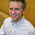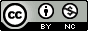## Wednesday, July 14, 2010

### QQ plot of p-values in R using base graphics

Update Tuesday, September 14, 2010: Fixed the ylim issue, now it sets the y axis limit based on the smallest observed p-value.

A while back Will showed you how to create QQ plots of p-values in Stata and in R using the now-deprecated sma package. A bit later on I showed you how to do the same thing in R using ggplot2. As much as we (and our readers) love ggplot2 around here, it can be quite a bit slower than using the built in base graphics. This was only recently a problem for me when I tried creating a quantile-quantile plot of over 12-million p-values. I wrote the code to do this in base graphics, which is substantially faster than using the ggplot2 code I posted a while back. The code an an example are below.

Here's what the resulting QQ-plot will look like:

1.Actually in base graphics, the easiest way to do the equangular line is abline(0,1, col='red')

2.Ah, thanks Abhijit. That'll might speed things up just slightly I imagine.

3.Also, for the qqplot, you really aren't plotting 1/n,2/n,...,1 against the p-values. There is a R function ppoints which gives the values against which the qqplot should be plotted. For large data this doesn't really matter, but technically....

Actually, you could just have done:
e = -log10(ppoints(10000))
o = -log10(pvalues)
qqplot(e, o, pch=19, cex=1, main=main, ...,
xlab=expression(Expected~~-log(italic(p))),
ylab=expression(Observed~~-log(italic(p))),
xlim=c(0,max(e)), ylim=c(0,max(e)))
abline(0,1, col='red')

If you wanted something quick and dirty you could just have done qqplot(ppoints(length(pvalues)), pvalues, log='xy') which would have done things in terms of log10(p) as opposed to -log10(p).

4.Shouldn't you be setting your y-limit to
ylim=c(0,max(o)) ?

Otherwise wouldn't it be cutting off everyone's "good news" p-values ??

5.Good catch Mike. This is now fixed. Thanks.

6.Stupid question, but why do you take the -log 10?

Note: Only a member of this blog may post a comment.